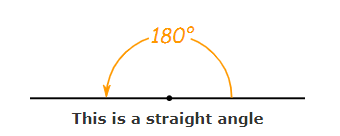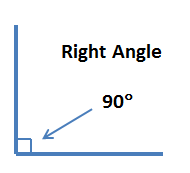# Free Introduction to Geometry 01 Practice Test - 4th Grade

We can say that the study of geometry starts with a ____.

A. line
B. point
C. angle
D. ray

#### SOLUTION

Solution : B

We can say that the study of geometry starts with a point. A point is an exact position in space. It is the basic unit of geometry. Any geometrical figure is a collection of infinite number of points.

A. shapes
B. songs
C. angles
D. lines

#### SOLUTION

Solution : A, C, and D

We study about points, lines, angles, shapes in geometry.
Songs are not taught in geometry.

When two lines cross each other at a point we call them ___ lines.

#### SOLUTION

Solution :

When two lines cross each other at a point we call them intersecting lines and the point where they meet is called the point of intersection.

Parallel lines never intersect each other.

We can find our way through a new city without knowing the directions.

A. False
B. True

#### SOLUTION

Solution : A

When visiting a new city or place, it would help to know the directions to guide us around. A map can be very useful. It lets us know where we are and can also help us identify directions that will help in navigating through a city.

The opposite edges of a desktop screen are  ___.

A. parallel lines
B. zigzag lines
C. vertical lines
D. diagonal lines

#### SOLUTION

Solution : AThe opposite edges of a desktop screen are always equally spaced and do not meet.

They are thus, examples of parallel lines.

A 90-degree angle is also called a ___ angle.

#### SOLUTION

Solution :

A 90-degree angle is also called a right angle.

A straight angle is ___ a reflex angle.

A. greater than
B. less than
C. equal to
D. bigger than

#### SOLUTION

Solution : B

A straight angle measures 180°.

A reflex angle measures more than a straight angle of 180°, but less than a full circle of 360°.

Hence, a straight angle is less than a reflex angle.

What is an angle that measures 360 called?

A. Whole angle
B. Obtuse angle
C. Straight angle
D. Right angle

#### SOLUTION

Solution : A

• An angle which measures 360 is  called a whole angle.• An angle which meaures greater than 180 and less than 270 is called an obtuse angle.• An angle which measures 180 is called a straight angle.• An angle which measures 90 is called a right angle.What are lines that are always at the same distance from each other and never intersect called?

A. Horizontal lines
B. Parallel lines
C. Intersecting lines
D. Vertical lines

#### SOLUTION

Solution : BWhen two (or more) lines are always at the same distance from each other and never intersect each other, they are called parallel lines.

The railway lines are examples of parallel lines.

How many right angles make a straight angle?

A. 1
B. 2
C. 3
D. 4

#### SOLUTION

Solution : B

A right angle is equal to 90 degrees.

A straight angle is equal to 180 degrees.

90×2=180

Hence, two right angles make a straight angle.